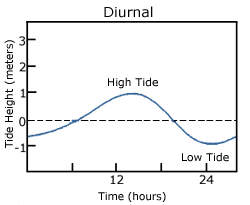# 11.3: Tide Classification

$$\newcommand{\vecs}{\overset { \rightharpoonup} {\mathbf{#1}} }$$ $$\newcommand{\vecd}{\overset{-\!-\!\rightharpoonup}{\vphantom{a}\smash {#1}}}$$$$\newcommand{\id}{\mathrm{id}}$$ $$\newcommand{\Span}{\mathrm{span}}$$ $$\newcommand{\kernel}{\mathrm{null}\,}$$ $$\newcommand{\range}{\mathrm{range}\,}$$ $$\newcommand{\RealPart}{\mathrm{Re}}$$ $$\newcommand{\ImaginaryPart}{\mathrm{Im}}$$ $$\newcommand{\Argument}{\mathrm{Arg}}$$ $$\newcommand{\norm}{\| #1 \|}$$ $$\newcommand{\inner}{\langle #1, #2 \rangle}$$ $$\newcommand{\Span}{\mathrm{span}}$$ $$\newcommand{\id}{\mathrm{id}}$$ $$\newcommand{\Span}{\mathrm{span}}$$ $$\newcommand{\kernel}{\mathrm{null}\,}$$ $$\newcommand{\range}{\mathrm{range}\,}$$ $$\newcommand{\RealPart}{\mathrm{Re}}$$ $$\newcommand{\ImaginaryPart}{\mathrm{Im}}$$ $$\newcommand{\Argument}{\mathrm{Arg}}$$ $$\newcommand{\norm}{\| #1 \|}$$ $$\newcommand{\inner}{\langle #1, #2 \rangle}$$ $$\newcommand{\Span}{\mathrm{span}}$$$$\newcommand{\AA}{\unicode[.8,0]{x212B}}$$

With so many variables playing a role in the production of tides, it is understandable that not every place on Earth will experience exactly the same tidal conditions. There are three primary classifications for tides, depending on the number and relative heights of tidal cycles per day.

A diurnal tide consists of only one high tide and one low tide per day (Figure $$\PageIndex{1}$$). “Diurnal” refers to a daily occurrence, so a situation where there is only one complete tidal cycle per day is considered a diurnal tide. Diurnal tides are common in the Gulf of Mexico, along the west coast of Alaska, and in parts of Southeast Asia.Figure $$\PageIndex{1}$$ A diurnal tide, with one high and one low tide per day (By NOAA [Public domain], via Wikimedia Commons).

A semidiurnal tide exhibits two high and two low tides each day, with both highs and both lows of toughly equal height (Figure $$\PageIndex{2}$$). “Semidiurnal” means “half of a day”; one tidal cycle takes half of a day, therefore there are two complete cycles per day. Semidiurnal tides are common along the east coasts of North America and Australia, the west coast of Africa, and most of Europe.Figure $$\PageIndex{2}$$ A semi-diurnal tide, with two high and two low tides per day, each of roughly equal heights (By NOAA [Public domain], via Wikimedia Commons).

Mixed semidiurnal tides (or mixed tides), have two high tides and two low tides per day, but the heights of each tide differs; the two high tides are of different heights, as are the two low tides (Figure $$\PageIndex{3}$$). The differences in height may be the result of amphidromic circulation, the angle of the moon, or any of the other variables discussed in section 11.2. Mixed semidiurnal tides are found along the Pacific coast of North America.Figure $$\PageIndex{3}$$ A mixed semi-diurnal tide, with two high and two low tides per day, each with a different height (By NOAA [Public domain], via Wikimedia Commons).

Figure $$\PageIndex{4}$$ shows the distribution of the various tide types throughout the world.Figure $$\PageIndex{4}$$ Global distribution of the different types of tides (By KVDP (Own work) [Public domain], via Wikimedia Commons).

## Tidal Currents

The movement of water with the rising and falling tide creates tidal currents. As the tide rises, water flows into an area, creating a flood current. As the tide falls and water flows out an ebb current is created. Slack water, or slack tides occur during the transition between incoming high and outgoing low tides, when there is no net water movement.

The strength of a tidal current depends on the volume of water that enters and exits with each tidal cycle (the tidal volume or tidal prism), and the area through which the water flows. A large tidal volume moving through a large area may create only a weak tidal current, as the volume is spread over a wide area. On the other hand, a narrow area may produce a strong tidal current even if the tidal volume is small, as all of the water is forced through a small area. It follows that the strongest tidal currents will result from a large tidal range moving through a narrow area.

Tidal bores occur where rivers meet the ocean. If the incoming tidal current is stronger than the river outflow, the tidal bore appears as a wave, or moving wall of water that moves up the river as the tide comes in (Figure $$\PageIndex{5}$$).Figure $$\PageIndex{5}$$ A tidal bore near Silverdale in the United Kingdom (Arnold Price [CC BY-SA 2.0], via Wikimedia Commons).

In many cases these tidal bores may move through a river or inlet for many kilometers, and if they are large enough they can form continually breaking waves that surfers can ride much farther and longer than a traditional ocean wave, such as the Severn Bore in England, shown in the video below.